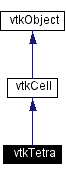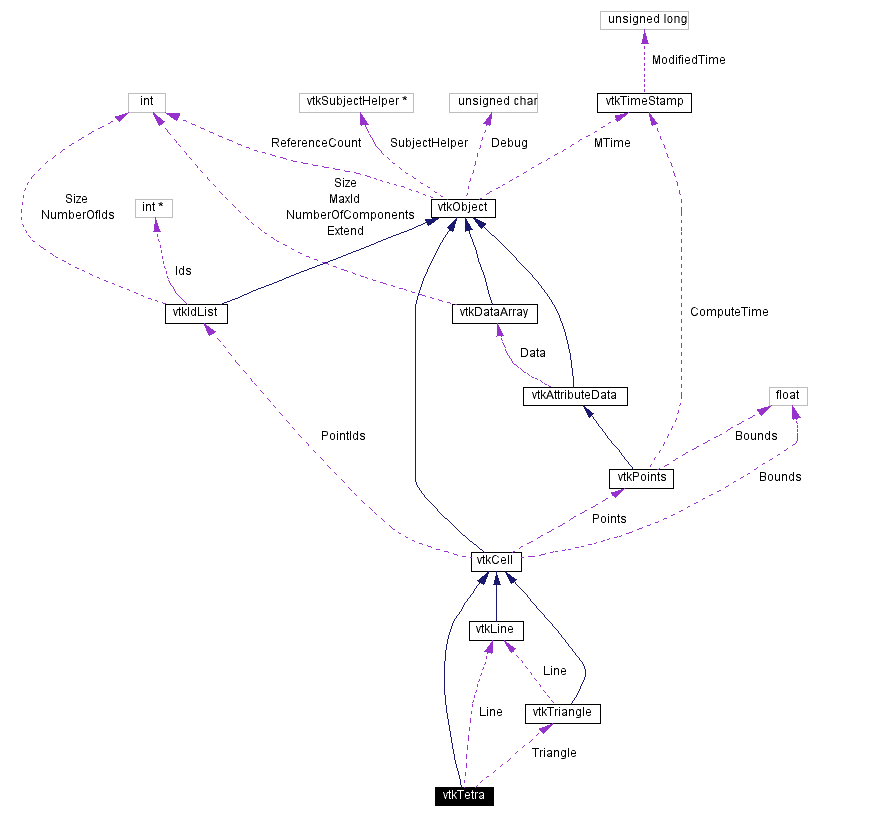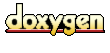Main Page   Class Hierarchy   Alphabetical List   Compound List   File List   Compound Members   File Members   Related Pages

# vtkTetra Class Reference

a 3D cell that represents a tetrahedron. More...

`#include <vtkTetra.h>`

Inheritance diagram for vtkTetra:[legend]
Collaboration diagram for vtkTetra:[legend]
List of all members.

## Public Methods

virtual const char * GetClassName ()
virtual int IsA (const char *type)
vtkCellMakeObject ()
int GetCellType ()
int GetCellDimension ()
int GetNumberOfEdges ()
int GetNumberOfFaces ()
vtkCellGetEdge (int edgeId)
vtkCellGetFace (int faceId)
void Contour (float value, vtkScalars *cellScalars, vtkPointLocator *locator, vtkCellArray *verts, vtkCellArray *lines, vtkCellArray *polys, vtkPointData *inPd, vtkPointData *outPd, vtkCellData *inCd, int cellId, vtkCellData *outCd)
int EvaluatePosition (float x, float *closestPoint, int &subId, float pcoords, float &dist2, float *weights)
void EvaluateLocation (int &subId, float pcoords, float x, float *weights)
int IntersectWithLine (float p1, float p2, float tol, float &t, float x, float pcoords, int &subId)
int Triangulate (int index, vtkIdList *ptIds, vtkPoints *pts)
void Derivatives (int subId, float pcoords, float *values, int dim, float *derivs)
int CellBoundary (int subId, float pcoords, vtkIdList *pts)
void Clip (float value, vtkScalars *cellScalars, vtkPointLocator *locator, vtkCellArray *tetras, vtkPointData *inPd, vtkPointData *outPd, vtkCellData *inCd, int cellId, vtkCellData *outCd, int insideOut)
int GetParametricCenter (float pcoords)
int JacobianInverse (double **inverse, float derivs)
int CellBoundary (int subId, float pcoords, vtkIdList &pts)
int Triangulate (int index, vtkIdList &ptIds, vtkPoints &pts)

## Static Public Methods

vtkTetra * New ()
int IsTypeOf (const char *type)
vtkTetra * SafeDownCast (vtkObject *o)
int * GetFaceArray (int faceId)
void TetraCenter (float p1, float p2, float p3, float p4, float center)
double Circumsphere (double p1, double p2, double p3, double p4, double center)
int BarycentricCoords (double x, double x1, double x2, double x3, double x4, double bcoords)
void InterpolationFunctions (float pcoords, float weights)
void InterpolationDerivs (float derivs)

## Protected Methods

vtkTetra ()
~vtkTetra ()
vtkTetra (const vtkTetra &)
void operator= (const vtkTetra &)

## Protected Attributes

vtkLineLine
vtkTriangleTriangle

## Detailed Description

a 3D cell that represents a tetrahedron.

Date:
2000/12/10 20:08:19
Revision:
1.56

vtkTetra is a concrete implementation of vtkCell to represent a 3D tetrahedron.

Examples:
vtkTetra (examples)

Definition at line 62 of file vtkTetra.h.

## Constructor & Destructor Documentation

 vtkTetra::vtkTetra ( ) ` [protected]`

 vtkTetra::~vtkTetra ( ) ` [protected]`

 vtkTetra::vtkTetra ( const vtkTetra & ) ` [inline, protected]`
 Definition at line 153 of file vtkTetra.h.

## Member Function Documentation

 vtkTetra* vtkTetra::New ( ) ` [static]`
 Create an object with Debug turned off, modified time initialized to zero, and reference counting on. Reimplemented from vtkObject.

 virtual const char* vtkTetra::GetClassName ( ) ` [virtual]`
 Return the class name as a string. This method is defined in all subclasses of vtkObject with the vtkTypeMacro found in vtkSetGet.h. Reimplemented from vtkCell.

 int vtkTetra::IsTypeOf ( const char * type ) ` [static]`
 Return 1 if this class type is the same type of (or a subclass of) the named class. Returns 0 otherwise. This method works in combination with vtkTypeMacro found in vtkSetGet.h. Reimplemented from vtkCell.

 virtual int vtkTetra::IsA ( const char * type ) ` [virtual]`
 Return 1 if this class is the same type of (or a subclass of) the named class. Returns 0 otherwise. This method works in combination with vtkTypeMacro found in vtkSetGet.h. Reimplemented from vtkCell.

 vtkTetra* vtkTetra::SafeDownCast ( vtkObject * o ) ` [static]`
 Will cast the supplied object to vtkObject* is this is a safe operation (i.e., a safe downcast); otherwise NULL is returned. This method is defined in all subclasses of vtkObject with the vtkTypeMacro found in vtkSetGet.h. Reimplemented from vtkCell.

 vtkCell* vtkTetra::MakeObject ( ) ` [virtual]`
 See the vtkCell API for descriptions of these methods. Reimplemented from vtkCell.

 int vtkTetra::GetCellType ( ) ` [inline, virtual]`
 Return the type of cell. Reimplemented from vtkCell. Definition at line 70 of file vtkTetra.h.

 int vtkTetra::GetCellDimension ( ) ` [inline, virtual]`
 Return the topological dimensional of the cell (0,1,2, or 3). Reimplemented from vtkCell. Definition at line 71 of file vtkTetra.h.

 int vtkTetra::GetNumberOfEdges ( ) ` [inline, virtual]`
 Return the number of edges in the cell. Reimplemented from vtkCell. Definition at line 72 of file vtkTetra.h.

 int vtkTetra::GetNumberOfFaces ( ) ` [inline, virtual]`
 Return the number of faces in the cell. Reimplemented from vtkCell. Definition at line 73 of file vtkTetra.h.

 vtkCell* vtkTetra::GetEdge ( int edgeId ) ` [virtual]`
 Return the edge cell from the edgeId of the cell. Reimplemented from vtkCell.

 vtkCell* vtkTetra::GetFace ( int faceId ) ` [virtual]`
 Return the face cell from the faceId of the cell. Reimplemented from vtkCell.

 int* vtkTetra::GetFaceArray ( int faceId ) ` [static]`

 void vtkTetra::Contour ( float value, vtkScalars * cellScalars, vtkPointLocator * locator, vtkCellArray * verts, vtkCellArray * lines, vtkCellArray * polys, vtkPointData * inPd, vtkPointData * outPd, vtkCellData * inCd, int cellId, vtkCellData * outCd ) ` [virtual]`
 Generate contouring primitives. The scalar list cellScalars are scalar values at each cell point. The point locator is essentially a points list that merges points as they are inserted (i.e., prevents duplicates). Contouring primitives can be vertices, lines, or polygons. It is possible to interpolate point data along the edge by providing input and output point data - if outPd is NULL, then no interpolation is performed. Also, if the output cell data is non-NULL, the cell data from the contoured cell is passed to the generated contouring primitives. (Note: the CopyAllocate() method must be invoked on both the output cell and point data. The cellId refers to the cell from which the cell data is copied.) Reimplemented from vtkCell.

 int vtkTetra::EvaluatePosition ( float x, float * closestPoint, int & subId, float pcoords, float & dist2, float * weights ) ` [virtual]`
 Given a point x return inside(=1) or outside(=0) cell; evaluate parametric coordinates, sub-cell id (!=0 only if cell is composite), distance squared of point x to cell (in particular, the sub-cell indicated), closest point on cell to x (unless closestPoint is null, in which case, the closest point and dist2 are not found), and interpolation weights in cell. (The number of weights is equal to the number of points defining the cell). Note: on rare occasions a -1 is returned from the method. This means that numerical error has occurred and all data returned from this method should be ignored. Also, inside/outside is determine parametrically. That is, a point is inside if it satisfies parametric limits. This can cause problems for cells of topological dimension 2 or less, since a point in 3D can project onto the cell within parametric limits but be "far" from the cell. Thus the value dist2 may be checked to determine true in/out. Reimplemented from vtkCell.

 void vtkTetra::EvaluateLocation ( int & subId, float pcoords, float x, float * weights ) ` [virtual]`
 Determine global coordinate (x) from subId and parametric coordinates. Also returns interpolation weights. (The number of weights is equal to the number of points in the cell.) Reimplemented from vtkCell.

 int vtkTetra::IntersectWithLine ( float p1, float p2, float tol, float & t, float x, float pcoords, int & subId ) ` [virtual]`
 Intersect with a ray. Return parametric coordinates (both line and cell) and global intersection coordinates, given ray definition and tolerance. The method returns non-zero value if intersection occurs. Reimplemented from vtkCell.

 int vtkTetra::Triangulate ( int index, vtkIdList * ptIds, vtkPoints * pts ) ` [virtual]`
 Generate simplices of proper dimension. If cell is 3D, tetrahedron are generated; if 2D triangles; if 1D lines; if 0D points. The form of the output is a sequence of points, each n+1 points (where n is topological cell dimension) defining a simplex. The index is a parameter that controls which triangulation to use (if more than one is possible). If numerical degeneracy encountered, 0 is returned, otherwise 1 is returned. Reimplemented from vtkCell.

 void vtkTetra::Derivatives ( int subId, float pcoords, float * values, int dim, float * derivs ) ` [virtual]`
 Compute derivatives given cell subId and parametric coordinates. The values array is a series of data value(s) at the cell points. There is a one-to-one correspondence between cell point and data value(s). Dim is the number of data values per cell point. Derivs are derivatives in the x-y-z coordinate directions for each data value. Thus, if computing derivatives for a scalar function in a hexahedron, dim=1, 8 values are supplied, and 3 deriv values are returned (i.e., derivatives in x-y-z directions). On the other hand, if computing derivatives of velocity (vx,vy,vz) dim=3, 24 values are supplied ((vx,vy,vz)1, (vx,vy,vz)2, ....()8), and 9 deriv values are returned ((d(vx)/dx),(d(vx)/dy),(d(vx)/dz), (d(vy)/dx),(d(vy)/dy), (d(vy)/dz), (d(vz)/dx),(d(vz)/dy),(d(vz)/dz)). Reimplemented from vtkCell.

 int vtkTetra::CellBoundary ( int subId, float pcoords, vtkIdList * pts ) ` [virtual]`
 Returns the set of points that are on the boundary of the tetrahedron that are closest parametrically to the point specified. This may include faces, edges, or vertices. Reimplemented from vtkCell.

 void vtkTetra::Clip ( float value, vtkScalars * cellScalars, vtkPointLocator * locator, vtkCellArray * tetras, vtkPointData * inPd, vtkPointData * outPd, vtkCellData * inCd, int cellId, vtkCellData * outCd, int insideOut ) ` [virtual]`
 Clip this tetra using scalar value provided. Like contouring, except that it cuts the tetra to produce other tetrahedra. Reimplemented from vtkCell.

 int vtkTetra::GetParametricCenter ( float pcoords ) ` [inline, virtual]`
 Return the center of the tetrahedron in parametric coordinates. Reimplemented from vtkCell. Definition at line 161 of file vtkTetra.h.

 void vtkTetra::TetraCenter ( float p1, float p2, float p3, float p4, float center ) ` [static]`
 Compute the center of the tetrahedron,

 double vtkTetra::Circumsphere ( double p1, double p2, double p3, double p4, double center ) ` [static]`
 Compute the circumcenter (center) and radius (method return value) of a tetrahedron defined by the four points x1, x2, x3, and x4.

 int vtkTetra::BarycentricCoords ( double x, double x1, double x2, double x3, double x4, double bcoords ) ` [static]`
 Given a 3D point x, determine the barycentric coordinates of the point. Barycentric coordinates are a natural coordinate system for simplices that express a position as a linear combination of the vertices. For a tetrahedron, there are four barycentric coordinates (because there are four vertices), and the sum of the coordinates must equal 1. If a point x is inside a simplex, then all four coordinates will be strictly positive. If three coordinates are zero (so the fourth =1), then the point x is on a vertex. If two coordinates are zero, the point x is on an edge (and so on). In this method, you must specify the vertex coordinates x1->x4. Returns 0 if tetrahedron is degenerate.

 int vtkTetra::JacobianInverse ( double ** inverse, float derivs )
 Given parametric coordinates compute inverse Jacobian transformation matrix. Returns 9 elements of 3x3 inverse Jacobian plus interpolation function derivatives. Returns 0 if no inverse exists.

 void vtkTetra::InterpolationFunctions ( float pcoords, float weights ) ` [static]`
 Tetra specific methods.

 void vtkTetra::InterpolationDerivs ( float derivs ) ` [static]`

 int vtkTetra::CellBoundary ( int subId, float pcoords, vtkIdList & pts ) ` [inline]`
 For legacy compatibility. Do not use. Definition at line 144 of file vtkTetra.h.

 int vtkTetra::Triangulate ( int index, vtkIdList & ptIds, vtkPoints & pts ) ` [inline]`
 Definition at line 146 of file vtkTetra.h.

 void vtkTetra::operator= ( const vtkTetra & ) ` [inline, protected]`
 Definition at line 154 of file vtkTetra.h.

## Member Data Documentation

 vtkLine* vtkTetra::Line` [protected]`
 Definition at line 156 of file vtkTetra.h.

 vtkTriangle* vtkTetra::Triangle` [protected]`
 Definition at line 157 of file vtkTetra.h.

The documentation for this class was generated from the following file:

Generated on Wed Nov 21 13:00:46 2001 for VTK by1.2.11.1 written by Dimitri van Heesch, © 1997-2001